Q-Chem 5.1 User’s Manual

# 4.5 Converging SCF Calculations

As for any numerical optimization procedure, the rate of convergence of the SCF procedure is dependent on the initial guess and on the algorithm used to step towards the stationary point. Q-Chem features a number of SCF optimization algorithms which can be selected via the $rem variable SCF_ALGORITHM, including: Methods that are based on extrapolation/interpolation: • The highly successful DIIS procedures. These are the default (except for restricted open-shell SCF calculations) and are available for all orbital types (see Section 4.5.3). • ADIIS (the Accelerated DIIS algorithm developed by Hu et al., available for R and U only). Methods that make use of orbital gradient: • Direct Minimization (DM), which has been re-implemented as simple steepest descent with line search, and is available for all orbital types. DM can be invoked after a few DIIS iterations. • Geometric Direct Minimization (GDM) which is an improved and highly robust version of DM and is the recommended fall-back when DIIS fails. Like DM, It can also be invoked after a few iterations with DIIS to improve the initial guess. GDM is the default algorithm for restricted open-shell SCF calculations and is available for all orbital types (see Section 4.5.4). • GDM_LS (it is essentially a preconditioned (using orbital energy differences as the preconditioner) L-BFGS algorithm with line search, available for R, U, RO and OS_RO). Methods that require orbital Hessian: • NEWTON_CG/NEWTON_MINRES (solve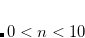for the update direction with CG/MINRES solvers). • SF_NEWTON_CG (the “saddle-free" version of NEWTON_CG). The analytical orbital Hessian is available for R/U/RO/G/CR unless special density functionals (e.g. those containing VV10) are used, while the use of finite-difference Hessian is available for all orbital types by setting FD_MAT_VEC_PROD = TRUE. In addition to these algorithms, there is also the maximum overlap method (MOM) which ensures that DIIS always occupies a continuous set of orbitals and does not oscillate between different occupancies. MOM can also be used to obtain higher-energy solutions of the SCF equations (see Section 7.4). The relaxed constraint algorithm (RCA), which guarantees that the energy goes down at every step, is also available via the old SCF code (set GEN_SCFMAN = FALSE). Nevertheless, the performance of the ADIIS algorithm should be similar to it. Since the code in GEN_SCFMAN is highly modular, the availability of different SCF algorithms to different SCF (orbital) types is largely extended in general. For example, the old ROSCF implementation requires the use of the GWH guess and the GDM algorithm exclusively. Such a limitation has been eliminated in GEN_SCFMAN based RO calculations. ## 4.5.2 Basic Convergence Control Options See also more detailed options in the following sections, and note that the SCF convergence criterion and the integral threshold must be set in a compatible manner, (this usually means THRESH should be set to at least 3 higher than SCF_CONVERGENCE). MAX_SCF_CYCLES  Controls the maximum number of SCF iterations permitted. TYPE:  INTEGER DEFAULT:  50 OPTIONS: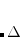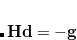User-selected. RECOMMENDATION:  Increase for slowly converging systems such as those containing transition metals. SCF_ALGORITHM  Algorithm used for converging the SCF. TYPE:  STRING DEFAULT:  DIIS Pulay DIIS. OPTIONS:  DIIS Pulay DIIS. DM Direct minimizer. DIIS_DM Uses DIIS initially, switching to direct minimizer for later iterations (See THRESH_DIIS_SWITCH, MAX_DIIS_CYCLES). DIIS_GDM Use DIIS and then later switch to geometric direct minimization (See THRESH_DIIS_SWITCH, MAX_DIIS_CYCLES). GDM Geometric Direct Minimization. RCA Relaxed constraint algorithm RCA_DIIS Use RCA initially, switching to DIIS for later iterations (see THRESH_RCA_SWITCH and MAX_RCA_CYCLES described later in this chapter) ROOTHAAN Roothaan repeated diagonalization. RECOMMENDATION:  Use DIIS unless performing a restricted open-shell calculation, in which case GDM is recommended. If DIIS fails to find a reasonable approximate solution in the initial iterations, RCA_DIIS is the recommended fallback option. If DIIS approaches the correct solution but fails to finally converge, DIIS_GDM is the recommended fallback. SCF_CONVERGENCE  SCF is considered converged when the wave function error is less that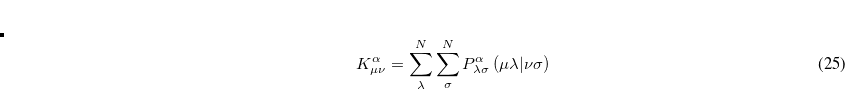. Adjust the value of THRESH at the same time. Note as of Q-Chem 3.0 the DIIS error is measured by the maximum error rather than the RMS error. TYPE:  INTEGER DEFAULT:  5 For single point energy calculations. 7 For geometry optimizations and vibrational analysis. 8 For SSG calculations, see Chapter 6. OPTIONS:Corresponding to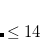RECOMMENDATION:  Tighter criteria for geometry optimization and vibration analysis. Larger values provide more significant figures, at greater computational cost. In some cases besides the total SCF energy, one needs its separate energy components, like kinetic energy, exchange energy, correlation energy, etc. The values of these components are printed at each SCF cycle if one specifies SCF_PRINT = 1 in the input. ## 4.5.3 Direct Inversion in the Iterative Subspace (DIIS) The SCF implementation of the Direct Inversion in the Iterative Subspace (DIIS) method uses the property of an SCF solution that requires the density matrix to commute with the Fock matrix: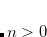(4.33) During the SCF cycles, prior to achieving self-consistency, it is therefore possible to define an error vector e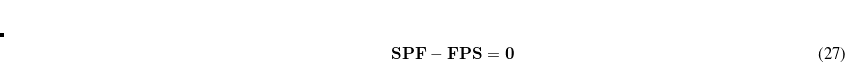, which is non-zero except at convergence: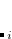(4.34) Here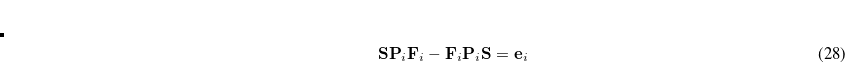is obtained by diagonalizing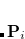, and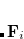(4.35) The DIIS coefficients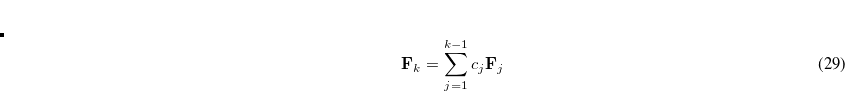, are obtained by a least-squares constrained minimization of the error vectors, viz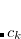(4.36) where the constraint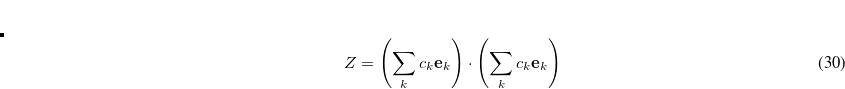is imposed to yield a set of linear equations, of dimension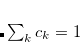: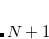(4.37) Convergence criteria require the largest element of the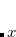th error vector to be below a cutoff threshold, usually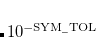a.u. for single point energies, but often increased to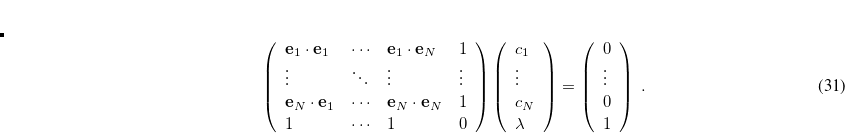a.u. for optimizations and frequency calculations. The rate of convergence may be improved by restricting the number of previous Fock matrices (size of the DIIS subspace,$rem variable DIIS_SUBSPACE_SIZE) used for determining the DIIS coefficients: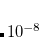(4.38)

where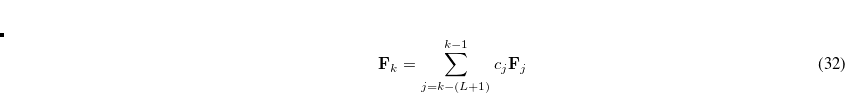is the size of the DIIS subspace. As the Fock matrix nears self-consistency, the linear matrix equations in Eq. (4.37) tend to become severely ill-conditioned and it is often necessary to reset the DIIS subspace (this is automatically carried out by the program).

Finally, on a practical note, we observe that DIIS has a tendency to converge to global minima rather than local minima when employed for SCF calculations. This seems to be because only at convergence is the density matrix in the DIIS iterations idempotent. On the way to convergence, one is not on the true energy surface, and this seems to permit DIIS to “tunnel” through barriers in wave function space. This is usually a desirable property, and is the motivation for the options that permit initial DIIS iterations before switching to direct minimization to converge to the minimum in difficult cases.

The following $rem variables permit some customization of the DIIS iterations: DIIS_SUBSPACE_SIZE  Controls the size of the DIIS and/or RCA subspace during the SCF. TYPE:  INTEGER DEFAULT:  15 OPTIONS:  User-defined RECOMMENDATION:  None DIIS_PRINT  Controls the output from DIIS SCF optimization. TYPE:  INTEGER DEFAULT:  0 OPTIONS:  0 Minimal print out. 1 Chosen method and DIIS coefficients and solutions. 2 Level 1 plus changes in multipole moments. 3 Level 2 plus Multipole moments. 4 Level 3 plus extrapolated Fock matrices. RECOMMENDATION:  Use the default Note: In Q-Chem 3.0 the DIIS error is determined by the maximum error rather than the RMS error. For backward compatibility the RMS error can be forced by using the following$rem

DIIS_ERR_RMS
 Changes the DIIS convergence metric from the maximum to the RMS error.

TYPE:
 LOGICAL

DEFAULT:
 FALSE

OPTIONS:
 TRUE, FALSE

RECOMMENDATION:
 Use the default, the maximum error provides a more reliable criterion.

DIIS_SEPARATE_ERRVEC
 Control optimization of DIIS error vector in unrestricted calculations.

TYPE:
 LOGICAL

DEFAULT:
 FALSE Use a combined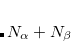and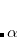error vector.

OPTIONS:
 FALSE Use a combinedanderror vector. TRUE Use separate error vectors for theandspaces.

RECOMMENDATION:
 When using DIIS in Q-Chem a convenient optimization for unrestricted calculations is to sum theanderror vectors into a single vector which is used for extrapolation. This is often extremely effective, but in some pathological systems with symmetry breaking, can lead to false solutions being detected, where theandcomponents of the error vector cancel exactly giving a zero DIIS error. While an extremely uncommon occurrence, if it is suspected, set DIIS_SEPARATE_ERRVEC = TRUE to check.

## 4.5.4 Geometric Direct Minimization (GDM)

Troy Van Voorhis, working at Berkeley with Martin Head-Gordon, has developed a novel direct minimization method that is extremely robust, and at the same time is only slightly less efficient than DIIS. This method is called geometric direct minimization (GDM) because it takes steps in an orbital rotation space that correspond properly to the hyper-spherical geometry of that space. In other words, rotations are variables that describe a space which is curved like a many-dimensional sphere. Just like the optimum flight paths for airplanes are not straight lines but great circles, so too are the optimum steps in orbital rotation space. GDM takes this correctly into account, which is the origin of its efficiency and its robustness. For full details, we refer the reader to Ref. VanVoorhis:2002a. GDM is a good alternative to DIIS for SCF jobs that exhibit convergence difficulties with DIIS.

Recently, Barry Dunietz, also working at Berkeley with Martin Head-Gordon, has extended the GDM approach to restricted open-shell SCF calculations. Their results indicate that GDM is much more efficient than the older direct minimization method (DM).

In section 4.5.3, we discussed the fact that DIIS can efficiently head towards the global SCF minimum in the early iterations. This can be true even if DIIS fails to converge in later iterations. For this reason, a hybrid scheme has been implemented which uses the DIIS minimization procedure to achieve convergence to an intermediate cutoff threshold. Thereafter, the geometric direct minimization algorithm is used. This scheme combines the strengths of the two methods quite nicely: the ability of DIIS to recover from initial guesses that may not be close to the global minimum, and the ability of GDM to robustly converge to a local minimum, even when the local surface topology is challenging for DIIS. This is the recommended procedure with which to invoke GDM (i.e., setting SCF_ALGORITHM = DIIS_GDM). This hybrid procedure is also compatible with the SAD guess, while GDM itself is not, because it requires an initial guess set of orbitals. If one wishes to disturb the initial guess as little as possible before switching on GDM, one should additionally specify MAX_DIIS_CYCLES = 1 to obtain only a single Roothaan step (which also serves up a properly orthogonalized set of orbitals).

The MOM-related $rem variables in full are the following:. MOM_PRINT  Switches printing on within the MOM procedure. TYPE:  LOGICAL DEFAULT:  FALSE OPTIONS:  FALSE Printing is turned off TRUE Printing is turned on. RECOMMENDATION:  None MOM_START  Determines when MOM is switched on to stabilize DIIS iterations. TYPE:  INTEGER DEFAULT:  0 (FALSE) OPTIONS:  0 (FALSE) MOM is not usedMOM begins on cycle. RECOMMENDATION:  Set to 1 if preservation of initial orbitals is desired. If MOM is to be used to aid convergence, an SCF without MOM should be run to determine when the SCF starts oscillating. MOM should be set to start just before the oscillations. MOM_METHOD  Determines the target orbitals with which to maximize the overlap on each SCF cycle. TYPE:  INTEGER DEFAULT:  3 OPTIONS:  3 Maximize overlap with the orbitals from the previous SCF cycle. 13 Maximize overlap with the initial guess orbitals. RECOMMENDATION:  If appropriate guess orbitals can be obtained, then MOM_METHOD = 13 can provide more reliable convergence to the desired solution. ## 4.5.7 Relaxed Constraint Algorithm (RCA) The relaxed constraint algorithm (RCA) is an ingenious and simple means of minimizing the SCF energy that is particularly effective in cases where the initial guess is poor. The latter is true, for example, when employing a user-specified basis (when the “core” or GWH guess must be employed) or when near-degeneracy effects imply that the initial guess will likely occupy the wrong orbitals relative to the desired converged solution. Briefly, RCA begins with the SCF problem as a constrained minimization of the energy as a function of the density matrix,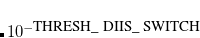. The constraint is that the density matrix be idempotent,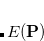, which basically forces the occupation numbers to be either zero or one. The fundamental realization of RCA is that this constraint can be relaxed to allow sub-idempotent density matrices,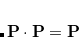. This condition forces the occupation numbers to be between zero and one. Physically, we expect that any state with fractional occupations can lower its energy by moving electrons from higher energy orbitals to lower ones. Thus, if we solve for the minimum ofsubject to the relaxed sub-idempotent constraint, we expect that the ultimate solution will nonetheless be idempotent. In fact, for Hartree-Fock this can be rigorously proven. For density functional theory, it is possible that the minimum will have fractional occupation numbers but these occupations have a physical interpretation in terms of ensemble DFT. The reason the relaxed constraint is easier to deal with is that it is easy to prove that a linear combination of sub-idempotent matrices is also sub-idempotent as long as the linear coefficients are between zero and one. By exploiting this property, convergence can be accelerated in a way that guarantees the energy will go down at every step. The implementation of RCA in Q-Chem closely follows the “Energy DIIS” implementation of the RCA algorithm. Here, the current density matrix is written as a linear combination of the previous density matrices: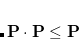(4.39) To a very good approximation (exact for Hartree-Fock) the energy for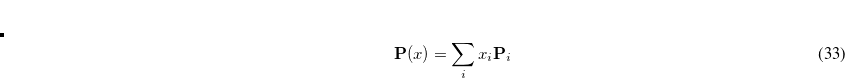can be written as a quadratic function of x: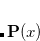(4.40) At each iteration,is chosen to minimize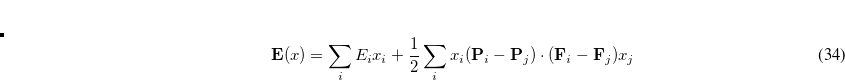subject to the constraint that all of the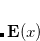are between zero and one. The Fock matrix foris further written as a linear combination of the previous Fock matrices,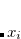(4.41) where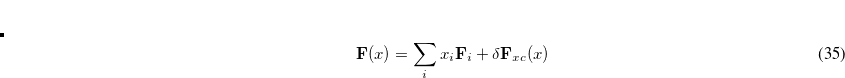denotes a (usually quite small) change in the exchange-correlation part that is computed oncehas been determined. We note that this extrapolation is very similar to that used by DIIS. However, this procedure is guaranteed to reduce the energyat every iteration, unlike DIIS. In practice, the RCA approach is ideally suited to difficult convergence situations because it is immune to the erratic orbital swapping that can occur in DIIS. On the other hand, RCA appears to perform relatively poorly near convergence, requiring a relatively large number of steps to improve the precision of a good approximate solution. It is thus advantageous in many cases to run RCA for the initial steps and then switch to DIIS either after some specified number of iterations or after some target convergence threshold has been reached. Finally, note that by its nature RCA considers the energy as a function of the density matrix. As a result, it cannot be applied to restricted open shell calculations which are explicitly orbital-based. Note: RCA interacts poorly with INCDFT, so INCDFT is disabled by default when an RCA or RCA_DIIS calculation is requested. To enable INCDFT with such a calculation, set INCDFT = 2 in the$rem section. RCA may also have poor interactions with incremental Fock builds; if RCA fails to converge, setting INCFOCK = FALSE may improve convergence in some cases.

Job-control variables for RCA are listed below, and an example input can be found in Section 4.5.11.

RCA_PRINT
 Controls the output from RCA SCF optimizations.

TYPE:
 INTEGER

DEFAULT:
 0

OPTIONS:
 0 No print out 1 RCA summary information 2 Level 1 plus RCA coefficients 3 Level 2 plus RCA iteration details

RECOMMENDATION:
 None

MAX_RCA_CYCLES
 The maximum number of RCA iterations before switching to DIIS when SCF_ALGORITHM is RCA_DIIS.

TYPE:
 INTEGER

DEFAULT:
 50

OPTIONS:
 N N RCA iterations before switching to DIIS

RECOMMENDATION:
 None

THRESH_RCA_SWITCH
 The threshold for switching between RCA and DIIS when SCF_ALGORITHM is RCA_DIIS.

TYPE:
 INTEGER

DEFAULT:
 3

OPTIONS:
 N Algorithm changes from RCA to DIIS when Error is less than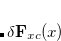.

RECOMMENDATION:
 None

## 4.5.8 User-Customized Hybrid SCF Algorithm

It is often the case that a single algorithm is not able to guarantee SCF convergence. Meanwhile, some SCF algorithms (e.g. ADIIS) can accelerate convergence at the beginning of an SCF calculation but becomes less efficient near the convergence. While a few hybrid algorithms (DIIS_GDM, RCA_DIIS) have been enabled in Q-Chem’s original SCF implementation, in GEN_SCFMAN, we seek for a more flexible setup for the use of multiple SCF algorithms so that users can have a more precise control on the SCF procedure. With the current implementation, at most four distinct algorithms (usually more than enough) can be employed in one single SCF calculation based on GEN_SCFMAN, and the basic job control is as follows:

GEN_SCFMAN_HYBRID_ALGO
 Use multiple algorithms in an SCF calculation based on GEN_SCFMAN.

TYPE:
 BOOLEAN

DEFAULT:
 FALSE

OPTIONS:
 FALSE Use a single SCF algorithm (given by SCF_ALGORITHM). TRUE Use multiple SCF algorithms (to be specified).

RECOMMENDATION:
 Set it to TRUE when the use of more than one algorithm is desired.

GEN_SCFMAN_ALGO_1
 The first algorithm to be used in a hybrid-algorithm calculation.

TYPE:
 STRING

DEFAULT:
 0

OPTIONS:
 All the available SCF_ALGORITHM options, including the GEN_SCFMAN additions (Section 4.3.1).

RECOMMENDATION:
 None

GEN_SCFMAN_ITER_1
 Maximum number of iterations given to the first algorithm. If used up, switch to the next algorithm.

TYPE:
 INTEGER

DEFAULT:
 50

OPTIONS:
 User-defined

RECOMMENDATION:
 None

GEN_SCFMAN_CONV_1
 The convergence criterion given to the first algorithm. If reached, switch to the next algorithm.

TYPE:
 INTEGER

DEFAULT:
 0

OPTIONS:10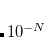RECOMMENDATION:
 None

Note: $rem variables GEN_SCFMAN_ALGO_X, GEN_SCFMAN_ITER_X, GEN_SCFMAN_CONV_X (X = 2, 3, 4) are defined and used in a similar way. Example 4.29 B3LYP/3-21G calculation for a cadmium-imidazole complex using the ADIIS-DIIS algorithm (an example from Ref. Hu:2010). Due to the poor quality of the CORE guess, using a single algorithm such as DIIS or GDM fails to converge. $molecule
2 1
Cd     0.000000     0.000000     0.000000
N      0.000000     0.000000    -2.260001
N     -0.685444     0.000000    -4.348035
C      0.676053     0.000000    -4.385069
C      1.085240     0.000000    -3.091231
C     -1.044752     0.000000    -3.060220
H      1.231530     0.000000    -5.300759
H      2.088641     0.000000    -2.711077
H     -2.068750     0.000000    -2.726515
H     -1.313170     0.000000    -5.174718
$end$rem
JOBTYPE                  SP
EXCHANGE                 B3LYP
BASIS                    3-21g
UNRESTRICTED             FALSE
SYMMETRY                 FALSE
SYM_IGNORE               TRUE
THRESH                   14
SCF_GUESS                CORE
GEN_SCFMAN               TRUE
GEN_SCFMAN_HYBRID_ALGO   TRUE
GEN_SCFMAN_CONV_1        3  !switch to DIIS when error < 1E-3
GEN_SCFMAN_ITER_1        50
GEN_SCFMAN_ALGO_2        DIIS
GEN_SCFMAN_CONV_2        8
GEN_SCFMAN_ITER_2        50
$end  ## 4.5.9 Internal Stability Analysis and Automated Correction for Energy Minima At convergence, the SCF energy will be at a stationary point with respect to changes in the MO coefficients. However, this stationary point is not guaranteed to be an energy minimum, and in cases where it is not, the wave function is said to be unstable. Even if the wave function is at a minimum, this minimum may be an artifact of the constraints placed on the form of the wave function. For example, an unrestricted calculation will usually give a lower energy than the corresponding restricted calculation, and this can give rise to an RHF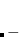UHF instability. Based on our experience, even for very simple data set such as the G2 atomization energies, using the default algorithm (DIIS) produces unstable solutions for several species (even for single atoms with some density functionals). In such cases, failure to check the internal stability of SCF solutions can result in flawed benchmark results. Although in general the use of gradient-based algorithms such as GDM is more likely to locate the true minimum, it still cannot entirely eliminate the possibility of finding an unstable solution. To understand what instabilities can occur, it is useful to consider the most general form possible for the spin orbitals: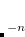(4.42) Here,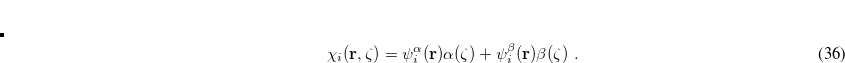and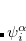are complex-valued functions of the Cartesian coordinates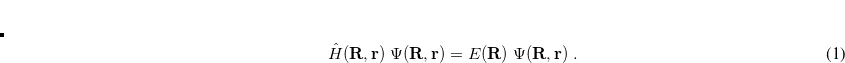, andandare spin eigenfunctions of the spin-variable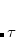. The first constraint that is almost universally applied is to assume the spin orbitals depend only on one or other of the spin-functionsor. Thus, the spin-functions take the form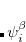(4.43) In addition, most SCF calculations use real functions, and this places an additional constraint on the form of the wave function. If there exists a complex solution to the SCF equations that has a lower energy, the wave function exhibits a realcomplex instability. The final constraint that is commonly placed on the spin-functions is that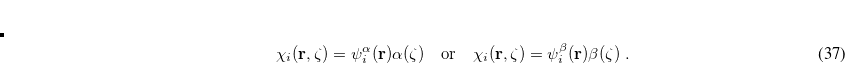, i.e., that the spatial parts of the spin-up and spin-down orbitals are the same. This gives the familiar restricted formalism and can lead to an RHFUHF instability as mentioned above. Further details about the possible instabilities can be found in Ref. Seeger:1977. Wave function instabilities can arise for several reasons, but frequently occur if • There exists a singlet diradical at a lower energy then the closed-shell singlet state. • There exists a triplet state at a lower energy than the lowest singlet state. • There are multiple solutions to the SCF equations, and the calculation has not found the lowest energy solution. Q-Chem’s previous stability analysis package suffered from the following limitations: • It is only available for restricted (close-shell) and unrestricted SCF calculations. • It requires the analytical orbital Hessian of the wave function energy. • The calculation terminates after the corrected MOs are generated, and a second job is needed to read in these orbitals and run another SCF calculation. The implementation of internal stability analysis in GEN_SCFMAN overcomes almost all these shortcomings. Its availability has been extended to all the implemented orbital types. As in the old code, when the analytical Hessian of the given orbital type and theory (e.g. RO/B3LYP) is available, it computes matrix-vector products analytically for the Davidson algorithm. If the analytical Hessian is not available, users can still run stability analysis by using the finite-difference matrix-vector product technique developed by Sharada et al., which requires the gradient (related to the Fock matrix) only: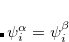(4.44) where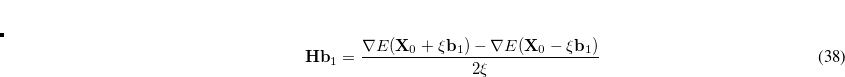is the Hessian matrix,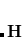is a trial vector,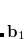stands for the current stationary point, and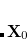is the finite step size. With this method, internal stability analysis is available for all the implemented orbital types in GEN_SCFMAN. It should be noted that since the second derivative of NLC functionals such as VV10 is not available in Q-Chem, this finite-difference method will be used by default for the evaluation of Hessian-vector products. GEN_SCFMAN allows multiple SCF calculations and stability analyses to be performed in a single job so that it can make use of the corrected MOs and locate the true minimum automatically. The MOs are displaced along the direction of the lowest-energy eigenvector (with line search) if an SCF solution is found to be unstable. A new SCF calculation that reads in these corrected MOs as initial guess will be launched automatically if INTERNAL_STABILITY_ITER > 0. Such macro-loops will keep going until a stable solution is reached. Note: The stability analysis package can be used to analyze both HF and DFT wave functions. ### 4.5.9.1 Job Control INTERNAL_STABILITY  Perform internal stability analysis in GEN_SCFMAN. TYPE:  BOOLEAN DEFAULT:  FALSE OPTIONS:  FALSE Do not perform internal stability analysis after convergence. TRUE Perform internal stability analysis and generate the corrected MOs. RECOMMENDATION:  Turn it on when the SCF solution is prone to unstable solutions, especially for open-shell species. FD_MAT_VEC_PROD  Compute Hessian-vector product using the finite difference technique. TYPE:  BOOLEAN DEFAULT:  FALSE (TRUE when the employed functional contains NLC) OPTIONS:  FALSE Compute Hessian-vector product analytically. TRUE Use finite difference to compute Hessian-vector product. RECOMMENDATION:  Set it to TRUE when analytical Hessian is not available. Note: For simple R and U calculations, it can always be set to FALSE, which indicates that only the NLC part will be computed with finite difference. INTERNAL_STABILITY_ITER  Maximum number of new SCF calculations permitted after the first stability analysis is performed. TYPE:  INTEGER DEFAULT:  0 (automatically set to 1 if INTERNAL_STABILITY = TRUE) OPTIONS:new SCF calculations permitted. RECOMMENDATION:  Give a larger number if 1 is not enough (still unstable). INTERNAL_STABILITY_DAVIDSON_ITER  Maximum number of Davidson iterations allowed in one stability analysis. TYPE:  INTEGER DEFAULT:  50 OPTIONS:Perform up toDavidson iterations. RECOMMENDATION:  Use the default. INTERNAL_STABILITY_CONV  Convergence criterion for the Davidson solver (for the lowest eigenvalues). TYPE:  INTEGER DEFAULT:  4 (3 when FD_MAT_ON_VECS = TRUE) OPTIONS:Terminate Davidson iterations when the norm of the residual vector is below 10. RECOMMENDATION:  Use the default. INTERNAL_STABILITY_ROOTS  Number of lowest Hessian eigenvalues to solve for. TYPE:  INTEGER DEFAULT:  2 OPTIONS:Solve forlowest eigenvalues. RECOMMENDATION:  Use the default. Example 4.30 Unrestricted SCF calculation of triplet B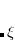using B97M-V/6-31g with the GDM algorithm. A displacement is performed when the first solution is characterized as a saddle point, and the second SCF gives a stable solution. $molecule
0 3
b
b 1 R

R = 1.587553
$end$rem
JOBTYPE              sp
METHOD               b97m-v
BASIS                6-31g
UNRESTRICTED         true
THRESH               14
SYMMETRY             false
SYM_IGNORE           true
SCF_FINAL_PRINT      1
SCF_ALGORITHM        gdm
SCF_CONVERGENCE      8
INTERNAL_STABILITY   true  !turn on internal stability analysis
FD_MAT_VEC_PROD      false !use finite-diff for the vv10 part only
$end  ## 4.5.10 Small-Gap Systems SCF calculations for systems with zero or small HOMO-LUMO gap (such as metals) can exhibit very slow convergence or may even fail to converge. This problem arises because the energetic ordering of orbitals and states can switch during the SCF optimization leading to discontinuities in the optimization. Using fractional MO occupation numbers can improve the convergence for small-gap systems. In this approach, the occupation numbers of MOs around the Fermi level are allowed to assume non-integer values. This “occupation smearing” allows one to include multiple electron configurations in the same optimization, which improves the stability of the optimization. We follow the pseudo-Fractional Occupation Number (pFON) method of Rabuck and Scuseria that scales the MO occupation used to construct the AO density: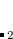(4.45) For a conventional (integer occupation number) SCF run, the occupation number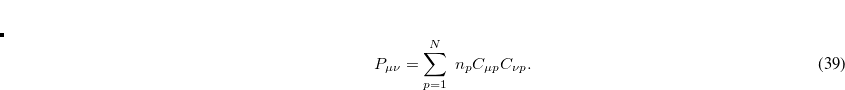is either one (occupied) or zero (virtual). In pFON, the occupation numbers are following a Fermi-Dirac distribution,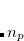(4.46) where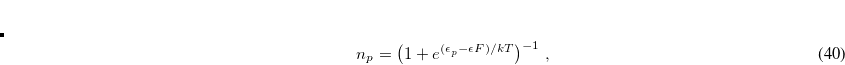is the respective orbital energy and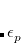the Boltzmann constant and temperature, respectively. The Fermi energy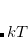is set to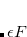in our implementation. To ensure conservation of the total number of electrons, the pFON approach re-scales the occupation numbers so that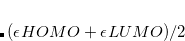. There are several parameters to control the electronic temperature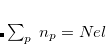throughout a pFON SCF run. The temperature can either be held constant at finite temperature (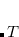=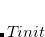), or the system can be cooled from a higher temperature down to the final temperature. So far, no zero-temperature extrapolation has been implemented. OCCUPATIONS  Activates pFON calculation. TYPE:  INTEGER DEFAULT:  0 OPTIONS:  0 Integer occupation numbers 1 Not yet implemented 2 Pseudo-fractional occupation numbers (pFON) RECOMMENDATION:  Use pFON to improve convergence for small-gap systems. FON_T_START  Initial electronic temperature (in K) for FON calculation. TYPE:  INTEGER DEFAULT:  1000 OPTIONS:  Any desired initial temperature. RECOMMENDATION:  Pick the temperature to either reproduce experimental conditions (e.g. room temperature) or as low as possible to approach zero-temperature. FON_T_END  Final electronic temperature for FON calculation. TYPE:  INTEGER DEFAULT:  0 OPTIONS:  Any desired final temperature. RECOMMENDATION:  Pick the temperature to either reproduce experimental conditions (e.g. room temperature) or as low as possible to approach zero-temperature. FON_NORB  Number of orbitals above and below the Fermi level that are allowed to have fractional occupancies. TYPE:  INTEGER DEFAULT:  4 OPTIONS:  n number of active orbitals RECOMMENDATION:  The number of valence orbitals is a reasonable choice. FON_T_SCALE  Determines the step size for the cooling. TYPE:  INTEGER DEFAULT:  90 OPTIONS:  n temperature is scaled by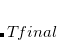in each cycle (cooling method 1) n temperature is decreased by n K in each cycle (cooling method 2) RECOMMENDATION:  The cooling rate should be neither too slow nor too fast. Too slow may lead to final energies that are at undesirably high temperatures. Too fast may lead to convergence issues. Reasonable choices for methods 1 and 2 are 98 and 50, respectively. When in doubt, use constant temperature. FON_E_THRESH  DIIS error below which occupations will be kept constant. TYPE:  INTEGER DEFAULT:  4 OPTIONS:  n freeze occupations below DIIS error ofRECOMMENDATION:  This should be one or two numbers bigger than the desired SCF convergence threshold. FON_T_METHOD  Selects cooling algorithm. TYPE:  INTEGER DEFAULT:  1 OPTIONS:  1 temperature is scaled by a factor in each cycle 2 temperature is decreased by a constant number in each cycle RECOMMENDATION:  We have made slightly better experience with a constant cooling rate. However, choose constant temperature when in doubt. ## 4.5.11 Examples Example 4.31 Input for a UHF calculation using geometric direct minimization (GDM) on the phenyl radical, after initial iterations with DIIS. $molecule
0   2
c1
x1  c1  1.0
c2  c1  rc2  x1  90.0
x2  c2  1.0  c1  90.0  x1    0.0
c3  c1  rc3  x1  90.0  c2    tc3
c4  c1  rc3  x1  90.0  c2   -tc3
c5  c3  rc5  c1   ac5  x1  -90.0
c6  c4  rc5  c1   ac5  x1   90.0
h1  c2  rh1  x2  90.0  c1  180.0
h2  c3  rh2  c1   ah2  x1   90.0
h3  c4  rh2  c1   ah2  x1  -90.0
h4  c5  rh4  c3   ah4  c1  180.0
h5  c6  rh4  c4   ah4  c1  180.0

rc2 =   2.672986
rc3 =   1.354498
tc3 =  62.851505
rc5 =   1.372904
ac5 = 116.454370
rh1 =   1.085735
rh2 =   1.085342
ah2 = 122.157328
rh4 =   1.087216
ah4 = 119.523496
$end$rem
BASIS           = 6-31G*
METHOD          = hf
SCF_ALGORITHM   = diis_gdm
SCF_CONVERGENCE = 7
THRESH          = 10
$end  Example 4.32 An example showing how to converge a ROHF calculation on the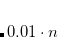state of DMX. Note the use of reading in orbitals from a previous closed-shell calculation and the use of MOM to maintain the orbital occupancies. The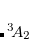is obtained if MOM is not used. $molecule
+1 1
C       0.000000     0.000000     0.990770
H       0.000000     0.000000     2.081970
C      -1.233954     0.000000     0.290926
C      -2.444677     0.000000     1.001437
H      -2.464545     0.000000     2.089088
H      -3.400657     0.000000     0.486785
C      -1.175344     0.000000    -1.151599
H      -2.151707     0.000000    -1.649364
C       0.000000     0.000000    -1.928130
C       1.175344     0.000000    -1.151599
H       2.151707     0.000000    -1.649364
C       1.233954     0.000000     0.290926
C       2.444677     0.000000     1.001437
H       2.464545     0.000000     2.089088
H       3.400657     0.000000     0.486785
$end$rem
UNRESTRICTED      false
METHOD            hf
BASIS             6-31+G*
SCF_GUESS         core
$end @@@$molecule
$end$rem
UNRESTRICTED      false
METHOD            hf
BASIS             6-31+G*
MOM_START         1
$end$occupied
1:26 28
1:26 28
$end @@@$molecule
-1 3
$end$rem
UNRESTRICTED      false
METHOD            hf
BASIS             6-31+G*
$end  Example 4.33 RCA_DIIS algorithm applied a radical $molecule
0 2
H    1.004123   -0.180454    0.000000
O   -0.246002    0.596152    0.000000
O   -1.312366   -0.230256    0.000000
$end$rem
UNRESTRICTED         true
METHOD               hf
BASIS                cc-pVDZ
SCF_GUESS            gwh
SCF_ALGORITHM        RCA_DIIS
THRESH               9
$end  Example 4.34 pFON calculation of a metal cluster. $molecule
0 1
Pt        -0.20408        1.19210        0.54029
Pt         2.61132        1.04687        0.66196
Pt         0.83227        0.03296       -1.49084
Pt         0.95832       -1.05360        0.92253
Pt        -1.66760       -1.07875       -1.02416
$end$rem
METHOD           pbe
ECP              fit-lanl2dz
SYMMETRY         false
OCCUPATIONS      2   ! pseudo-fractional occupation numbers
FON_NORB         10
FON_T_START      300 ! electronic temperature: 300 K
FON_T_END        300
FON_E_THRESH     5   ! freeze occupation numbers once DIIS error is 10^-5
\$end